## RD Sharma Class 10 Solutions Chapter 10 Trigonometric Ratios VSAQS

These Solutions are part of RD Sharma Class 10 Solutions. Here we have given RD Sharma Class 10 Solutions Chapter 10 Trigonometric Ratios VSAQS

Other Exercises

Answer each of the following questions either in one word or one sentence or as per requirement of the questions :

Question 1.
Write the maximum and minimum values of sin θ.
Solution:
Maximum value of sin θ = 1
and minimum value of sin θ = 0

Question 2.
Write the maximum and minimum values of cos 0.
Solution:
Maximum value cos θ=1 and minimum value of cos θ = θ

Question 3.
What is the maximum value of $$\frac { 1 }{ sec\theta }$$ ?
Solution:
Maximum value of $$\frac { 1 }{ sec\theta }$$ or cos θ = 1

Question 4.
What is the maximum value of $$\frac { 1 }{ cosec\theta }$$
Solution:
Maximum value of $$\frac { 1 }{ cosec\theta }$$ or sin θ = 1

Question 5.Solution:Question 6.Solution:Question 7.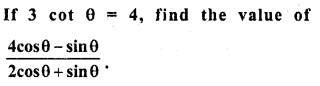Solution: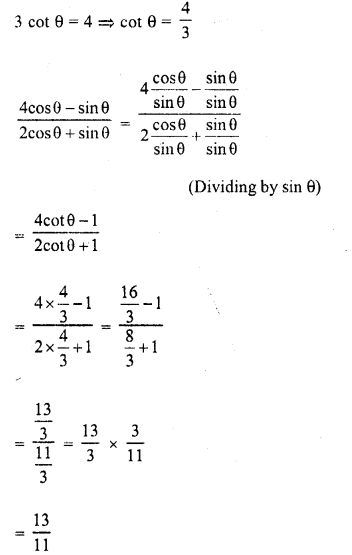Question 8.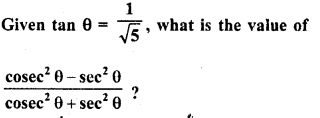Solution: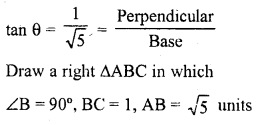Question 9.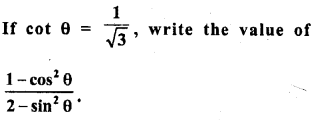Solution: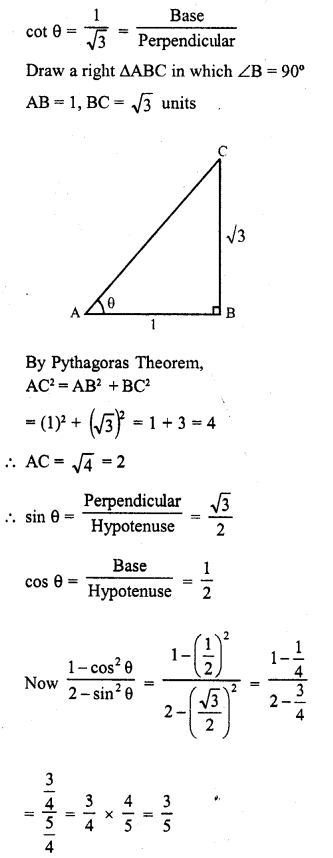Question 10.
If tan A = $$\frac { 3 }{ 4 }$$ and A + B = 90°, then what is the value of cot B ?
Solution: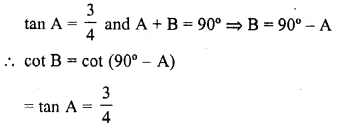Question 11.
If A + B = 90°, cos B = $$\frac { 3 }{ 5 }$$, what is the value of sin A.
Solution: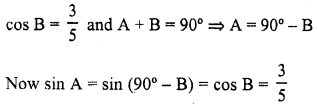Question 12.
Write the acute angle θ satisfying √3 sin θ = cos θ.
Solution:Question 13.
Write the Value of cos 1° cos 2° cos 3° ……. cos 179° cos 180°.
Solution:Question 14.
Write the Value of tan 10′ tan 15° tan 75° tan 80°.
Solution: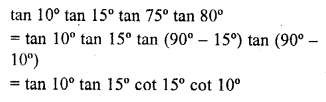Question 15.
If A + B = 90° and tan A = $$\frac { 3 }{ 4 }$$ what is cot B?
Solution: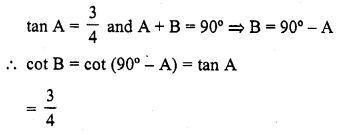Question 16.
If tan A = $$\frac { 5 }{ 12 }$$, find the value of (sin A + cos A) sec A. (C.B.S.E. 2008)
Solution: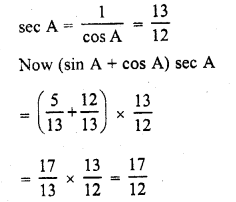Hope given RD Sharma Class 10 Solutions Chapter 10 Trigonometric Ratios VSAQS are helpful to complete your math homework.

If you have any doubts, please comment below. Learn Insta try to provide online math tutoring for you.